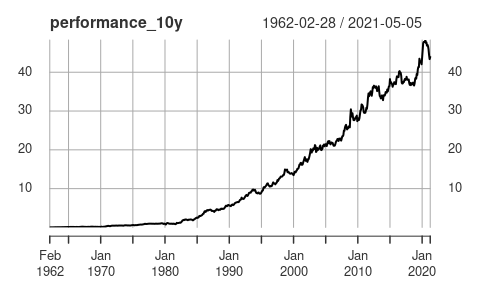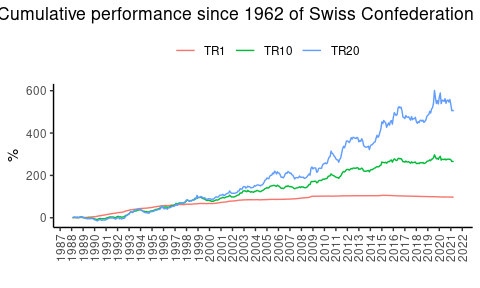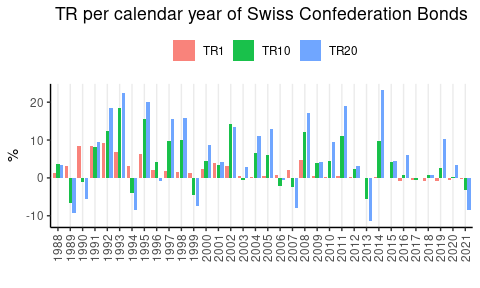# treasuryTR

#### 2021-05-07

The treasuryTR package offers the functionality to calculate the total return (TR) index from constant-maturity bond yields.

While Treasury yields are easy to come by1, total returns (TR) are not. The TR is what is earned by investors, and is therefore of paramount importance e.g. when simulating a treasury-stock diversified portfolio. A supplier for proprietary TR Treasury index data is CRSP2. Their data can be purchased or accesses trough a handful of commercial research platforms.

The TR can be computed from publicly available (constant-maturity) yield-to-maturity time-series data using standard (fixed-income) textbook formulas. Swinkels 2019 compares the TR series with alternative series (CRSP, Bloomberg etc.) and finds that the returns are very close and are therefore a high-quality alternative to commercially available data.

# Get yield data and compute TR

## Daily

get_yields() relies on quantmod::getSymbols() and can be used for downloading constant-maturity US treasury returns.

Common maturities are: DGS1MO 1-Month, DGS3MO: 3-Month, DGS6MO: 6-Month, DGS1 1-Year, DGS2 2-Year, DGS3 3-Year, DGS5 5-Year, DGS7 7-Year, DGS10 10-Year, DGS20 20-Year, and DGS30 30-Year Treasury Constant Maturity Rate.

library(treasuryTR)

yield_1y <- get_yields("DGS1")
Sys.sleep(1)
yield_10y <- get_yields("DGS10")
Sys.sleep(1)
yield_20y <- get_yields("DGS20")
Sys.sleep(1)

tr_1y <- total_return(yield_1y, maturity = 1, scale = 261)
tr_10y <- total_return(yield_10y, maturity = 10, scale = 261)
tr_20y <- total_return(yield_20y, maturity = 20, scale = 261)

#>                     DGS1         DGS10         DGS20
#> 1962-01-02            NA            NA            NA
#> 1962-01-03 -7.377978e-05  0.0026052037  0.0001528606
#> 1962-01-04  1.221768e-04  0.0034295689  0.0015146560
#> 1962-01-05 -7.300865e-05 -0.0022968905 -0.0012057347
#> 1962-01-08 -3.647573e-04 -0.0006649767 -0.0012041801
#> 1962-01-09  2.721722e-05 -0.0014782454  0.0001532288

library(PerformanceAnalytics)
table.AnnualizedReturns(cbind.xts(tr_1y, tr_10y, tr_20y), Rf = tr_1y, scale = 262)
#>                              DGS1  DGS10  DGS20
#> Annualized Return          0.0502 0.0662 0.0738
#> Annualized Std Dev         0.0120 0.0717 0.1027
#> Annualized Sharpe (Rf=NA%) 0.0000 0.2176 0.2237

In the example above, we get yields for the 1-Year, the 10-Year, and the 20-Year US treasury bonds. All of these yield series start in 1962. We calculate the TR using total_return(). It is worth noting that we use scale by 262, as this is the average number of days per year that the yield is reported on. It might be different for other data sources.

## Monthly

Swinkels (2019) compares the TR series he computes based on monthly 10-Year treasury yields to common treasury indices from CRSP, Global Financial Data, Ibbotson, and Bloomberg. He finds that the computed series are close to the reported indices.

yield_10y_monthly <- yield_10y[endpoints(yield_10y, on = "months", k = 1)]

tr_10y_monthly <- total_return(yield_10y_monthly, 10, scale = 12)

performance_10y <- cumprod(1+tr_10y_monthly[-1])-1

plot(performance_10y)table.AnnualizedReturns(tr_10y_monthly, scale = 12)
#>                            DGS10
#> Annualized Return         0.0663
#> Annualized Std Dev        0.0784
#> Annualized Sharpe (Rf=0%) 0.8456

## Dplyr style

The treasuryTR package also allows for use in dplyr-style syntax.

library(dplyr)

yield_10y_df <- get_yields("DGS10", format_out = "tibble")

tr_10y_df <- yield_10y_df %>%
mutate(TR = total_return(DGS10, maturity = 10))

tr_10y_df %>%
filter(!is.na(TR)) %>%
summarise(mu = mean(TR)*262,
sigma = sd(TR)*sqrt(262))
#> # A tibble: 1 x 2
#>       mu  sigma
#>    <dbl>  <dbl>
#> 1 0.0667 0.0717

Step-by-step calculation.

tr_10y_df_stepbystep <- yield_10y_df %>%
mutate(mod_duration = mod_duration(DGS10, 10),
convexity = convexity(DGS10, 10),
TR = total_return(DGS10, maturity = 10,
mdur = mod_duration,
convex = convexity))

tail(tr_10y_df_stepbystep)
#> # A tibble: 6 x 5
#>   date        DGS10 mod_duration convexity         TR
#>   <date>      <dbl>        <dbl>     <dbl>      <dbl>
#> 1 2021-04-28 0.0163         9.19      93.3  0.0000620
#> 2 2021-04-29 0.0165         9.18      93.2 -0.00177
#> 3 2021-04-30 0.0165         9.18      93.2  0.0000627
#> 4 2021-05-03 0.0163         9.19      93.3  0.00190
#> 5 2021-05-04 0.0161         9.20      93.4  0.00190
#> 6 2021-05-05 0.0159         9.21      93.6  0.00191

## Other data

Let’s use Swiss yield data that we download using the dataseries package.

library(dataseries)
library(tidyr)
library(ggplot2)

swiss_yields <- ds(c("ch_snb_rendoblim.1j",
"ch_snb_rendoblim.10j",
"ch_snb_rendoblim.20j"))

swiss_tr <- swiss_yields %>%
mutate(TR1 = total_return(ch_snb_rendoblim.1j/100, maturity = 1, scale = 12),
TR10 = total_return(ch_snb_rendoblim.10j/100, maturity = 10, scale = 12),
TR20 = total_return(ch_snb_rendoblim.20j/100, maturity = 20, scale = 12)) %>%
select(time, starts_with("TR")) %>%
pivot_longer(cols = -time) %>%
filter(!is.na(value)) %>%
arrange(name, time)

swiss_tr %>%
group_by(name) %>%
mutate(performance = cumprod(1+value)-1) %>%
ggplot(aes(x = time, y = performance*100, color = name)) +
geom_line() +
scale_y_continuous() +
scale_x_date(date_breaks = "1 year", date_labels = "%Y") +
labs(title = "Cumulative performance since 1962 of Swiss Confederation Bonds",
x = "", y = "%", color = "") +
theme_classic() +
theme(legend.position = "top",
plot.title = element_text(hjust = 0.5),
axis.text.x = element_text(angle = 90, vjust = 0.5))swiss_tr %>%
group_by(name, year = format(time, "%Y")) %>%
summarise(TR = prod(1+value)-1) %>%
ggplot(aes(x = year, y = TR*100, fill = name)) +
geom_col(position = position_dodge2(), alpha = 0.9) +
scale_y_continuous() +
labs(title = "TR per calendar year of Swiss Confederation Bonds",
x = "", y = "%", fill = "") +
theme_classic() +
theme(legend.position = "top",
plot.title = element_text(hjust = 0.5),
axis.text.x = element_text(angle = 90, vjust = 0.5),
panel.grid.major.x = element_line())
#> summarise() has grouped output by 'name'. You can override using the .groups argument.1. E.g. on the Federal Reserve Bank of St. Louis’s data portal “FRED”, see https://fred.stlouisfed.org/series/DGS5 (5 year), https://fred.stlouisfed.org/series/DGS10 (10 year), https://fred.stlouisfed.org/series/DGS20 (20 years), https://fred.stlouisfed.org/series/DGS30 (30 years)↩︎

2. Center for Research in Security Prices, LLC, see http://www.crsp.org/↩︎